Atomic Structure

# Atomic Structure

Test Description

## 30 Questions MCQ Test Chemistry Mock Test Series for JEE | Atomic Structure

Atomic Structure for Class 12 2023 is part of Chemistry Mock Test Series for JEE preparation. The Atomic Structure questions and answers have been prepared according to the Class 12 exam syllabus.The Atomic Structure MCQs are made for Class 12 2023 Exam. Find important definitions, questions, notes, meanings, examples, exercises, MCQs and online tests for Atomic Structure below.
Solutions of Atomic Structure questions in English are available as part of our Chemistry Mock Test Series for JEE for Class 12 & Atomic Structure solutions in Hindi for Chemistry Mock Test Series for JEE course. Download more important topics, notes, lectures and mock test series for Class 12 Exam by signing up for free. Attempt Atomic Structure | 30 questions in 45 minutes | Mock test for Class 12 preparation | Free important questions MCQ to study Chemistry Mock Test Series for JEE for Class 12 Exam | Download free PDF with solutions
 1 Crore+ students have signed up on EduRev. Have you?
Atomic Structure - Question 1

### Which statement relating to the spectrum of H atom is false?

Detailed Solution for Atomic Structure - Question 1

The spectral lines are closer only when ΔE  is large, i.e,λ is small

Atomic Structure - Question 2

### Two electrons A and B in an atom have the following set of quantum numbers : A :    3, 2, -2, +1/2,        B :    3, 0, 0, +1/2, Which statement is correct for A and B ?

Detailed Solution for Atomic Structure - Question 2

For A, (n+l) = 5 For B, (n+l) = 3.
Thus, larger is value of (n+l) more is energy level.

Atomic Structure - Question 3

### Which have the same number of s-electrons as the d-electrons in Fe2+ ?

Detailed Solution for Atomic Structure - Question 3

P has 6 electrons in s-subshells as in d-shell of Fe2+.

Atomic Structure - Question 4

The statements, which is/are correct:

Detailed Solution for Atomic Structure - Question 4

The desired formulae to calculate nodes.

Atomic Structure - Question 5

Which has highest e/m ratio?

Detailed Solution for Atomic Structure - Question 5

Mass of H+ is minimum.

Atomic Structure - Question 6

The ratio of e/m, i.e., specific charge for a cathode ray :

Detailed Solution for Atomic Structure - Question 6

A characteristic of cathode rays particles (electrons), e/m is constant as electron is fundamental particle

Atomic Structure - Question 7

Which is not permissble subshell?

Detailed Solution for Atomic Structure - Question 7

For n = 2; l can have value only 0 and 1, i.e., s and p-subshells.

Atomic Structure - Question 8

Non-directonal orbital is :

Detailed Solution for Atomic Structure - Question 8

s-orbitals being spherical and thus , non-directional.

Atomic Structure - Question 9

In hydrogen atom, which energy level order is not correct :

Detailed Solution for Atomic Structure - Question 9

In H atom, subshell of a shell posses same energy level, as energy depends only on value of ‘n’

Atomic Structure - Question 10

The n+l value for the 3p-energy level is :

Detailed Solution for Atomic Structure - Question 10

n = 3; l = 1

∴(n + l) = 4

Atomic Structure - Question 11

Which set has the same number of unpiared electrons in their ground state?

Detailed Solution for Atomic Structure - Question 11

N and P have 3 unpaired electrons in 2p and 3p respectively; V has 3 unpaired electrons in 3d.

Atomic Structure - Question 12

Which statement does not form part of Bohr’s model of the hydrogen atom?

Detailed Solution for Atomic Structure - Question 12

It represent Heisenberg’s uncertainly principle.

Atomic Structure - Question 13

Which best describe the emission spectra of atomic hydrogen?

Detailed Solution for Atomic Structure - Question 13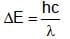Atomic Structure - Question 14

The statement are valid for :
(I)    in filling a group of orbitals of equal  energy it is energetically preferable to assign electron to empty orbitals rather than pair them into a particular orbital.
(II)    when two electrons are placed in two different orbitals, energy is lower if the spins are parallel.

Detailed Solution for Atomic Structure - Question 14

Only Hund’s rule discuss filling of electrons in degenerate orbitals.

Atomic Structure - Question 15

If E1,E2 and E3 represent respectively the kinetic energies of an electron, an alpha particle and a proton each having same de Broglie wavelength then :

Detailed Solution for Atomic Structure - Question 15

According to de broglie,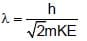Atomic Structure - Question 16

A quanta will have more energy, if :

Detailed Solution for Atomic Structure - Question 16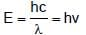Atomic Structure - Question 17

Which of the following statement is not correct?

Detailed Solution for Atomic Structure - Question 17

The energy level of an orbital in multi-electron system depends upon n & l.

Atomic Structure - Question 18

The angular speed of the electron in the nth orbit of Bohr hydrogen atom is :

Detailed Solution for Atomic Structure - Question 18

Angular speed is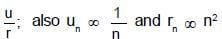Atomic Structure - Question 19

Angular momentum of an electron in an orbital is given by :

Detailed Solution for Atomic Structure - Question 19

It is expression to represent angular momentum of an electron in an orbital.

Atomic Structure - Question 20

When atoms are bombarded with α-particles, only a few in million of the α-particles suffer deflections, while others pass through undeflected.

Detailed Solution for Atomic Structure - Question 20

It is a fact derived by Rutherford from his a α- scattering experiment.

Atomic Structure - Question 21

Electronic configuration of H- is :

Detailed Solution for Atomic Structure - Question 21

H- has two electrons.

Atomic Structure - Question 22

What is the correct orbital designation for the electron with the quantum number, n = 4, m = -2, s = 1/2?

Detailed Solution for Atomic Structure - Question 22

l = 3 represent for f-subshell.

Atomic Structure - Question 23

The ratio of nucleons in O16 and O18 is :

Detailed Solution for Atomic Structure - Question 23

The no. of nucleons in O16 and O18 are 16 and 18 respectively.

Atomic Structure - Question 24

Photoelectric emission is observed from a surfcae for frequency v1 and v2 of the incident radiation (v1 > v2). If the maximum kinetic ebergies of the photoelectrons in the two cases are in the ratio 1:k, then the threshold frequency v0 is given by :

Detailed Solution for Atomic Structure - Question 24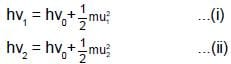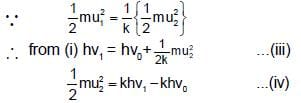By eqs. (ii) and (iv),
hv2 = hv0-khv0+khv1
or v0(1-k) = v2-kv1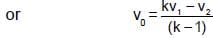Atomic Structure - Question 25

The radii of two of the first four Bohr’s orbits of the hydrogen atom are in the ratio 1 : 4. The energy difference between them may be:

Detailed Solution for Atomic Structure - Question 25

The two orbits are either I and II or II and IV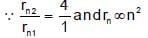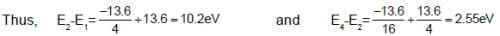Atomic Structure - Question 26

When photons of energy 4.25eV strike the surface of a metal A, the ejected photoelectrons have maximum kinetic energy, TA (expressed in eV) and de broglie wavelength,λA, The maximum kinetic energy of photoelectrons liberated from another metal B by photons of energy 4.70V is TB = TA - 1.50 eV. If the de Broglie wavelength of these photoelectrons is =,λB = 2λA then which is not correct?

Detailed Solution for Atomic Structure - Question 26

Let work function of A and B be wA and wB and TA, TB are kinetic energy
∴ 4.25 = wA + TA
or TA=4.25-wA
similarly TB=4.70-wB
∴ TB-TA=0.45+wA-wB
-1.5=0.45+wA-wB     (∴TB-TA=-1.5)
or   wB-wA= 1.95
∴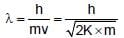∴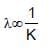(K is kinetic energy)
∴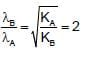Also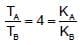∴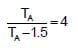∴   TA= 2eV   TB= 0.5eV   wA = 2.25 eV   wB = 4.2 eV

Atomic Structure - Question 27

Which ion has the maximum magnetic moment?

Detailed Solution for Atomic Structure - Question 27

Magnetic moment =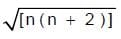where n is number of unpaired electrons.

Atomic Structure - Question 28

The de Broglie wavelength of a particle with mass 1g and velocity 100m/s is:

Detailed Solution for Atomic Structure - Question 28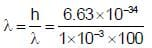= 6.63 * 10-33m

Atomic Structure - Question 29

The uncertainty in the momentum of an electron is 10-5 kg m s-1. The uncertainty in its position will be:

Detailed Solution for Atomic Structure - Question 29

Use ΔX.ΔP = h/4π

Atomic Structure - Question 30

The energy of second Bohr’s orbit in H-atom is -328 kJ mol-1, hence the energy of fourth Bohr orbit would be :

Detailed Solution for Atomic Structure - Question 30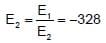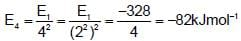## Chemistry Mock Test Series for JEE

1 docs|26 tests
 Use Code STAYHOME200 and get INR 200 additional OFF Use Coupon Code